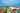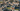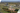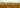# Colleges with the lowest SAT scores in District of Columbia

Top 6 colleges in District of Columbia with the lowest SAT scores
Looking for the colleges with the lowest SAT scores in District of Columbia? Well you're in luck! We've compiled a national college database and have created a list of the top 6 universities with the lowest SAT scores in District of Columbia below. If you are not a good test taker or worried about your test scores, this list is for you. These are the schools whose applicants had the lowest average SAT scores in District of Columbia, which means that you can get into these colleges with a lower SAT score. We also include each college's ACT scores and acceptance rate so that you can see where you would have the easiest time getting in. Read on to find out more.

## Gallaudet University SAT scores

The average SAT score for Gallaudet University is 940.The average SAT score of 940 breaks down into:

• SAT math: 470

The average ACT score for Gallaudet University is 17 and their acceptance rate is 57.1%.

## Howard University SAT scores

The average SAT score for Howard University is 1213.The average SAT score of 1213 breaks down into:

• SAT math: 593

The average ACT score for Howard University is 25 and their acceptance rate is 31.6%.

## The Catholic University of America SAT scores

The average SAT score for The Catholic University of America is 1230.The average SAT score of 1230 breaks down into:

• SAT math: 610

The average ACT score for The Catholic University of America is 27 and their acceptance rate is 83.7%.

## American University SAT scores

The average SAT score for American University is 1300.The average SAT score of 1300 breaks down into:

• SAT math: 635

The average ACT score for American University is 29 and their acceptance rate is 31.5%.

## The George Washington University SAT scores

The average SAT score for The George Washington University is 1370.The average SAT score of 1370 breaks down into:

• SAT math: 690

The average ACT score for The George Washington University is 31 and their acceptance rate is 41.9%.

## Georgetown University SAT scores

The average SAT score for Georgetown University is 1450.The average SAT score of 1450 breaks down into:

• SAT math: 735